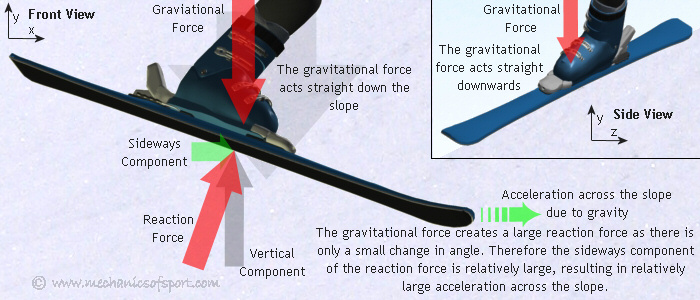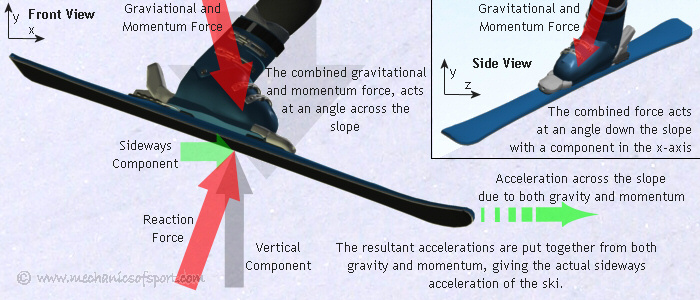SKIING

# Resistance Effects

The next thing we need to do is look at the effects created when a ski is at an angle to velocity and to the fall line. Whenever a ski is not sideways to a slope it creates a sideways component across the slope in the reaction force, which in turn creates an acceleration across the slope. The ski can still act with the same 4 edge effects, but it is the components of gravity and momentum in the opposite direction to the reaction force that cause these effects. If a ski is decelerating sideways, momentum is creating a force, and this force will not act straight down the slope like gravity, unless that is the direction you are traveling in. Therefore to work out the accelerations, the directions and magnitudes of the forces from both momentum and gravity must be taken into account. To start with we are going to look at the case where both gravity and momentum are acting straight down the slope, and then show what happens when momentum is in other directions.

## Gravity and Momentum in the Same Direction

In the diagram below, gravity and momentum are both acting straight down the slope.When momentum is not acting in the same direction down the slope as gravity though, it must be considered seperately, or by combining the forces and directions of gravity and momentum. This basically creates an equation that has 2 parts. The gravitational part, which determines whether the ski accelerates, decelerates or stays at a constant speed in the direction of the fall line, and the momentum part, which determines whether the ski keeps or loses its speed in the direction of its momentum. Remembering that both parts will create movement in other directions if the ski is not sideways to the forces. The ski can accelerate in the direction of momentum too, but the force that makes this happen has to come from gravity.

## Gravitational Component

Below we can see the gravity part of the equation, with a sideways acceleration being created from the reaction force.## Momentum Component

The next diagram shows the momentum part of the equation, with the force from momentum acting along the ski a lot more than the gravitational force.## Gravity and Momentum Combined

Below the diagram shows the result when both the gravitational and momentum parts of the equation are put together.Using the same ways of calculating forces as shown in the last section, what effects a ski will have at different angles to momentum and gravity can be worked out.

In the next section we learn how these forces and effects are used to give us our control while skiing, and what they enable us to do.

On to the Using Resistance section.